# SAT Math Multiple Choice Question 993: Answer and Explanation

### Test Information

Question: 993

13. If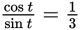, then what is tan t?

• A.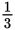• B.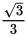• C.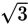• D. 3

Explanation:

D

Difficulty: Medium

Category: Additional Topics in Math / Trigonometry

Strategic Advice: A useful trig property to know is that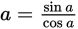.

Getting to the Answer: Given the ratio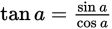, it follows that taking the reciprocal results in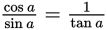. Thus, if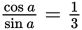, then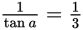. Again, taking the reciprocal yields tan a = 3, choice (D).

If you didn't know the property, you could also arrive at the answer by using SOH CAH TOA: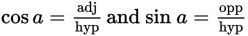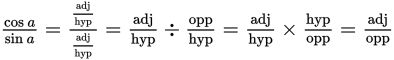Because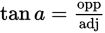, it follows from the above that tan a is the reciprocal of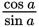.